# NCERT Solutions for Class 10 Maths Exercise 3.5## myCBSEguide App

Complete Guide for CBSE Students

NCERT Solutions, NCERT Exemplars, Revison Notes, Free Videos, CBSE Papers, MCQ Tests & more.

NCERT solutions for Maths Pair of Linear Equations in Two Variables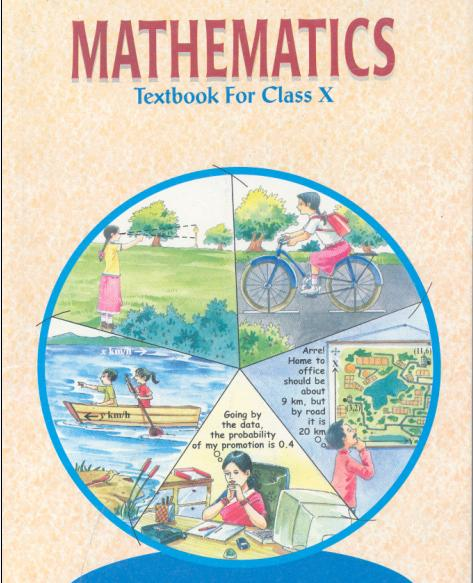## NCERT Solutions for Class 10 Maths Pair of Linear Equations in Two Variables

###### 1. Which of the following pairs of linear equations has unique solution, no solution, or infinitely many solutions? In case there is a unique solution, find it by using cross multiplication method.

(i) x – 3y – 3 = 0

3x – 9y – 2 = 0

(ii)2x + y = 5

3x + 2y = 8

(iii) 3x − 5y = 20

6x − 10y = 40

(iv) x − 3y – 7 = 0

3x − 3y – 15 = 0

Ans. (i) x − 3y – 3 = 0

3x − 9y – 2 = 0

Comparing equation x − 3y – 3 = 0 with a1x +b1y + c1 = 0 and 3x − 9y – 2 = 0 with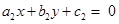,

We get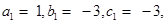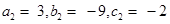Here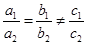this means that the two lines are parallel.

Therefore, there is no solution for the given equations i.e. it is inconsistent.

(ii) 2x + y = 5

3x + 2y = 8

Comparing equation 2x + y = 5 with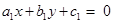and 3x + 2y = 8 with,

We get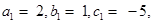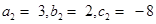###### Here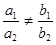this means that there is unique solution for the given equations.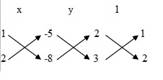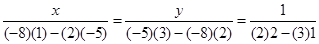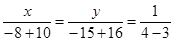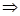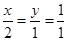x = 2 and y = 1

NCERT Solutions for Class 10 Maths Exercise 3.5

(iii) 3x − 5y = 20

6x − 10y = 40

Comparing equation 3x − 5y = 20 withand 6x − 10y = 40 with,

We get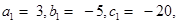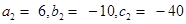Here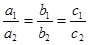It means lines coincide with each other.

Hence, there are infinite many solutions.

(iv) x − 3y – 7 = 0

3x − 3y – 15 = 0

Comparing equation x − 3y – 7 = 0 withand 3x − 3y – 15 = 0 with,

We get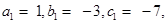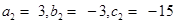Herethis means that we have unique solution for these equations.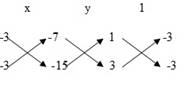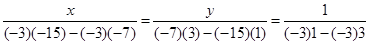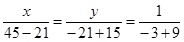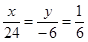x = 4 and y = –1

NCERT Solutions for Class 10 Maths Exercise 3.5

###### 2. (i) For which values of a and b does the following pair of linear equations have an

infinite number of solutions?

2x + 3y = 7

(a b) x + (a + b) y = 3a + b – 2

(ii) For which value of k will the following pair of linear equations have no

solution?

3x + y = 1

(2k − 1) x + (k − 1) y = 2k + 1

Ans. (i) Comparing equation 2x + 3y – 7 = 0 withand (a b) x + (a + b)

y − 3a b + 2 = 0 withWe get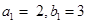and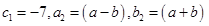and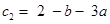###### Linear equations have infinite many solutions if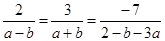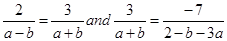⇒ 2a + 2b = 3a − 3b and 6 − 3b − 9a = −7a − 7b

a = 5b… (1) and −2a = −4b – 6… (2)

Putting (1) in (2), we get

−2 (5b) = −4b – 6

⇒ −10b + 4b = −6

⇒ −6b = –6 ⇒ b = 1

Putting value of b in (1), we get

a = 5b = 5 (1) = 5

Therefore, a = 5 and b = 1

(ii) Comparing (3x + y – 1 = 0) withand (2k − 1)x + (k − 1)y −2k – 1 = 0) with,

We get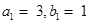and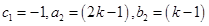and c2 = −2k − 1

Linear equations have no solution if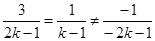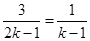⇒ 3 (k − 1) = 2k – 1

⇒ 3k – 3 = 2k − 1

k = 2

NCERT Solutions for Class 10 Maths Exercise 3.5

###### 3. Solve the following pair of linear equations by the substitution and cross-multiplication methods:

8x + 5y = 9

3x + 2y = 4

Ans. Substitution Method

8x + 5y = 9 … (1)

3x + 2y = 4 … (2)

From equation (1),

5y = 9 − 8xy =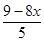NCERT Solutions for Class 10 Maths Exercise 3.5

Putting this in equation (2), we get

3x + 2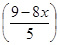= 4

⇒ 3x +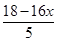= 4

⇒ 3x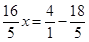⇒ 15x − 16x = 20 – 18

x = −2

Putting value of x in (1), we get

8 (−2) + 5y = 9

⇒ 5y = 9 + 16 = 25⇒ y = 5

Therefore, x = −2 and y = 5

###### Cross multiplication method

8x + 5y = 9 … (1)

3x + 2y = 4 … (2)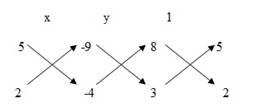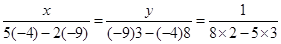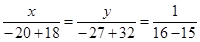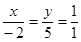x = −2 and y = 5

NCERT Solutions for Class 10 Maths Exercise 3.5

###### 4. Form the pair of linear equations in the following problems and find their solutions (if they exist) by any algebraic method:

(i) A part of monthly hostel charges is fixed and the remaining depends on the number of days one has taken food in the mess. When a student A takes food for 20 days she has to pay Rs 1000 as hostel charges whereas a student B, who takes food for 26 days, pays Rs 1180 as hostel charges. Find the fixed charges and the cost of food per day.

(ii) A fraction becomes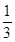when 1 is subtracted from the numerator and it becomes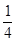when 8 is added to its denominator. Find the fraction.

(iii) Yash scored 40 marks in a test, getting 3 marks for each right answer and losing 1 mark for each wrong answer. Had 4 marks been awarded for each correct answer and 2 marks been deducted for each incorrect answer, then Yash would have scored 50 marks. How many questions were there in the test?

(iv) Places A and B are 100 km apart on a highway. One car starts from A and another from B at the same time. If the cars travel in the same direction at different speeds, they meet in 5 hours. If they travel towards each other, they meet in 1 hour. What are the speeds of the two cars?

(v) The area of a rectangle gets reduced by 9 square units, if its length is reduced by 5 units and breadth is increased by 3 units. If we increase the length by 3 units and the breadth by 2 units, the area increases by 67 square units. Find the dimensions of the rectangle.

Ans.(i)Let fixed monthly charge = Rs x and let charge of food for one day = Rs y

According to given conditions,

x + 20y = 1000 … (1),

and x + 26y = 1180 … (2)

Subtracting equation (1) from equation (2), we get

6y = 180

y = 30

Putting value of y in (1), we get

x + 20 (30) = 1000

x = 1000 – 600 = 400

Therefore, fixed monthly charges = Rs 400 and, charges of food for one day = Rs 30

(ii) Let numerator = x and let denominator = y

According to given conditions,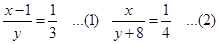⇒ 3x – 3 = y … (1) 4x = y + 8 … (1)

⇒ 3x y = 3 … (1) 4x y = 8 … (2)

Subtracting equation (1) from (2), we get

4x y − (3x y) = 8 – 3

x = 5

Putting value of x in (1), we get

3 (5) – y = 3

⇒ 15 – y = 3

y = 12

Therefore, numerator = 5 and, denominator = 12

It means fraction =

NCERT Solutions for Class 10 Maths Exercise 3.5

(iii) Let number of correct answers = x and let number of wrong answers = y

According to given conditions,

3x y = 40 … (1)

And, 4x − 2y = 50 … (2)

From equation (1), y = 3x − 40

Putting this in (2), we get

4x – 2 (3x − 40) = 50

⇒ 4x − 6x + 80 = 50

⇒ −2x = −30

x = 15

Putting value of x in (1), we get

3 (15) – y = 40

⇒ 45 – y = 40

y = 45 – 40 = 5

Therefore, number of correct answers = x = 15and number of wrong answers = y = 5

Total questions = x + y = 15 + 5 = 20

(iv)Let speed of car which starts from part A = x km/hr

Let speed of car which starts from part B = y km/hr

According to given conditions,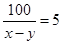(Assuming x > y)

⇒ 5x − 5y = 100

x y = 20 … (1)

And,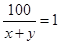x + y = 100 … (2)

Adding (1) and (2), we get

2x = 120

x = 60 km/hr

Putting value of x in (1), we get

60 – y = 20

y = 60 – 20 = 40 km/hr

Therefore, speed of car starting from point A = 60 km/hr

And, Speed of car starting from point B = 40 km/hr

(v) Let length of rectangle = x units and Let breadth of rectangle = y units

Area =xy square units. According to given conditions,

NCERT Solutions for Class 10 Maths Exercise 3.5

xy – 9 = (x − 5) (y + 3)

xy – 9 = xy + 3x − 5y – 15

⇒ 3x − 5y = 6 … (1)

And, xy + 67 = (x + 3) (y + 2)

xy + 67 = xy + 2x + 3y + 6

⇒ 2x + 3y = 61 … (2)

From equation (1),

3x = 6 + 5y

x =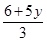Putting this in (2), we get

2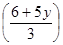+ 3y = 61

⇒ 12 + 10y + 9y = 183

⇒ 19y = 171

y = 9 units

Putting value of y in (2), we get

2x + 3 (9) = 61

⇒ 2x = 61 – 27 = 34

x = 17 units

Therefore, length = 17 units and, breadth = 9 units

## NCERT Solutions for Class 10 Maths Exercise 3.5

NCERT Solutions Class 10 Maths PDF (Download) Free from myCBSEguide app and myCBSEguide website. Ncert solution class 10 Maths includes text book solutions from Mathematics Book. NCERT Solutions for CBSE Class 10 Maths have total 15 chapters. 10 Maths NCERT Solutions in PDF for free Download on our website. Ncert Maths class 10 solutions PDF and Maths ncert class 10 PDF solutions with latest modifications and as per the latest CBSE syllabus are only available in myCBSEguide.

## CBSE app for Class 10

To download NCERT Solutions for Class 10 Maths, Computer Science, Home Science,Hindi ,English, Social Science do check myCBSEguide app or website. myCBSEguide provides sample papers with solution, test papers for chapter-wise practice, NCERT solutions, NCERT Exemplar solutions, quick revision notes for ready reference, CBSE guess papers and CBSE important question papers. Sample Paper all are made available through the best app for CBSE students and myCBSEguide website.## Test Generator

Create Papers with your Name & Logo

### 22 thoughts on “NCERT Solutions for Class 10 Maths Exercise 3.5”

1. nic app jisne bhi bnayi vo banda kitne kant hoga nic app ???????????????

2. These notes are same matches to my school notes

3. nice????

4. I love it
Good and ray to understand solutions
Truly GODLIKE!!!!!!!!!!!

6. Nice very simple method use i can easily understand

7. Nice very simple method use i can easily understand

9. I understand very easily, thak you

10. Very very very nice app I just like it

11. nice

12. exclent app and easy answer

13. Really it helps me

14. it really helps me
thanks a lot

15. I like this app very much ..if u r not use this app .. please use this I take bet from u that u will also like this app when u use those. …. I’m

16. Really very easy &good process It mathod very easy for us thank u so much

17. Nice.. Good..

18. east or west “my cbse guide” is the best

19. Very nice explanation of solutions.I really like it.

20. Wow?

21. Very good app shukriya

22. Excellent app